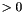## Apophenia

apop_mle_settings Struct Reference

## Data Fields

double delta

double dim_cycle_tolerance

int iters_fixed_T

double k

int max_iterations

char * method

double mu_t

int n_tries

apop_data ** path

gsl_rng * rng

double * starting_pt

double step_size

double t_initial

double t_min

double tolerance

int verbose

## Detailed Description

The settings for maximum likelihood estimation (including simulated annealing).

## Field Documentation

 double apop_mle_settings::dim_cycle_tolerance

If zero (the default), the usual procedure. If, cycle across dimensions: fix all but the first dimension at the starting point, optimize only the first dim. Then fix the all but the second dim, and optimize the second dim. Continue through all dims, until the log likelihood at the outset of one cycle through the dimensions is within this amount of the previous cycle's log likelihood. There will be at least two cycles.

 int apop_mle_settings::max_iterations

Ignored by simulated annealing. Other methods halt (and set the `"status"` element of the output estimate's info page) if they do this many iterations without finding an optimum.

 char * apop_mle_settings::method

The method to be used for the optimization. All strings are case-insensitive.

 String Name Notes "NM simplex" Nelder-Mead simplex Does not use gradients at all. Can sometimes get stuck. "FR cg" Conjugate gradient (Fletcher-Reeves) (default) CG methods use derivatives. The converge to the optimum of a quadratic function in one step; performance degrades as the objective digresses from quadratic. "BFGS cg" Broyden-Fletcher-Goldfarb-Shanno conjugate gradient "PR cg" Polak-Ribiere conjugate gradient "Annealing" simulated annealing Slow but works for objectives of arbitrary complexity, including stochastic objectives. "Newton" Newton's method Search by finding a root of the derivative. Expects that gradient is reasonably well-behaved. "Newton hybrid" Newton's method/gradient descent hybrid Find a root of the derivative via the Hybrid method If Newton proposes stepping outside of a certain interval, use an alternate method. See the GSL manual for discussion. "Newton hybrid no scale" Newton's method/gradient descent hybrid with spherical scale As above, but use a simplified trust region.
 apop_data ** apop_mle_settings::path

If not `NULL`, record each vector tried by the optimizer as one row of this apop_data set. Each row of the `matrix` element holds the vector tried; the corresponding element in the `vector` is the evaluated value at that vector (after out-of-constraints penalties have been subtracted). A new apop_data set is allocated at the pointer you send in. This data set has no names; add them as desired. For a sample use, see Optimization.

 double * apop_mle_settings::starting_pt

An array of doubles (e.g., `(double*){2,4,6,8}`) suggesting a starting point. If NULL, use an all-ones vector. If `startv` is a `gsl_vector` and is not a view of a matrix, use `.starting_pt=startv->data`.

 double apop_mle_settings::step_size

The initial step size.

 double apop_mle_settings::tolerance

The precision the minimizer uses in its stopping rule. Only vaguely related to the precision of the actual MLE.

 int apop_mle_settings::verbose

Give status updates as we go. This is orthogonal to the `apop_opts.verbose` setting.